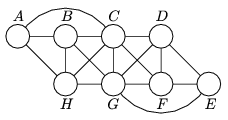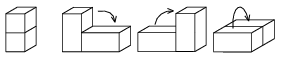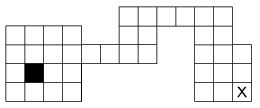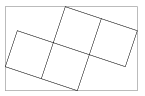Mathematical and Physical Journal
for High Schools
Issued by the MATFUND Foundation
 Already signed up? New to KöMaL?

# KöMaL Problems in Mathematics, October 2008

Show/hide problems of signs:## Problems with sign 'K'

Deadline expired on November 10, 2008.

K. 175. Write the numbers 1 to 8 in the eight circles of the Figure, so that no number is connected to the number one smaller or one larger (e.g. 4 is not connected to 3 or 5). Find all possible solutions. Two solutions are considered different if they contain different numbers in the circle marked with the same letter.(6 pont)

solution (in Hungarian), statistics

K. 176. Alex is playing the game called Bloxorz. It involves rolling a rectangular block on a board with a lattice of unit squares. The base of the block is a unit square and its height is two units. The block always covers one or two fields of the board. The first Figure shows the allowed moves of the block.In each move, the block is turned around an edge so that it lands on a face adjacent to the bottom. During the game, it is not allowed for the block to leave the board, that is, it cannot even partly overhang the edge. The purpose of the game is to move the block from the starting position to a standing position on the field marked X (that is, it should only touch that field of the board). (See http://miniclip.com/games/bloxorz/en for the internet version of the game.)

The second Figure shows the board. Initially, the block is standing on the black field. Move the block to the field marked X, in a standing position. Draw the successive intermediate positions of the block (indicating the order with numbers), through which it gets to the field marked X. Keep marking the positions on the same diagram until the next position overlaps with some previous position. Then draw a new diagram and continue to show further positions. Find a solution that consists of less than 30 steps.(6 pont)

solution (in Hungarian), statistics

K. 177. On what condition will the sum of the squares of six consecutive natural numbers be divisible by 7?

(6 pont)

solution (in Hungarian), statistics

K. 178. From the second term onwards, the nth term of a sequence of numbers is obtained by adding n to the reciprocal of the preceding term of the sequence. The fifth term is. Find the fourth and sixth terms.

(6 pont)

solution (in Hungarian), statistics

K. 179. Jack represented on a pie chart what he bought on his money in October. He spent 1500 forints (HUF, Hungarian currency) on lunch, 1000 forints on presents, 1250 forints on movie tickets, 500 forints on hiring films on DVD. His spendings on chewing gum were represented by a 20-degree sector of the pie chart. He had no other expenses in addition to those mentioned above. How much did he spend on chewing gum in October?

(6 pont)

solution (in Hungarian), statistics

K. 180. The numbers 1 to 9 are written in a 3×3 table in some order. The six three-digit numbers obtained by reading the rows left to right and the columns top to bottom are all added. What are the two smallest possible sums obtained in this way? For example, in the arrangement shown by the Figure, the sum obtained is 531+296+748+527+394+168=2664.

 5 3 1 2 9 6 7 4 8

(6 pont)

solution (in Hungarian), statistics## Problems with sign 'C'

Deadline expired on November 17, 2008.

C. 955. Solve the equation 10x-5=9[x] on the set of real numbers (where [x] stands for the greatest integer not greater than x).

(5 pont)

solution (in Hungarian), statistics

C. 956. The number of sides of a polygon is doubled. For what polygons will the sum of the angles of the new polygon be a multiple of the sum of the angles of the original polygon?

(5 pont)

solution (in Hungarian), statistics

C. 957. The sides of a rectangle are a and b. Two congruent circles are placed in a rectangle so that they have no interior point in common. What is the ratio of the sides of the rectangle if the diameter of the largest possible circles is?

(5 pont)

solution (in Hungarian), statistics

C. 958. In a certain board game played with a die, our token is four fields from home. If we roll at least four, we get home. If we roll a three, we can be certain that the next roll will take us home. What is the probability that we need more than two rolls to get home?

(5 pont)

solution (in Hungarian), statistics

C. 959. The diameter of one base of a truncated cone is 100 mm. If the diameter is increased by 21%, leaving the height and the size of the other base unchanged, the volume of the truncated cone will also increase by 21%. What is the diameter of the other base?

(5 pont)

solution (in Hungarian), statistics## Problems with sign 'B'

Deadline expired on November 17, 2008.

B. 4112. A billiards ball at corner A of a rectangular billiards table ABCD is struck. It starts moving along the angle bisector. It rebounds from the sides CD, BC and finally AB, and collides head-on with a ball that is at the centre of the rectangle. In what other direction is it possible to strike the ball at point A, so that it rebounds from three different sides and then hits the ball at the centre head-on?

(4 pont)

solution (in Hungarian), statistics

B. 4113. Prove that if a, b, c, d are integers and a+b+c+d=0 then 2(a4+b4+c4+d4)+8abcd is a square number.

(4 pont)

solution (in Hungarian), statistics

B. 4114. Four congruent squares are cut out of an 11 by 7 rectangle as shown in the Figure.

What percentage of the rectangle falls off?(3 pont)

solution (in Hungarian), statistics

B. 4115. For what positive integers k does 1 occur as a term of the sequence (an), given that a1=k andif an is even, and an+1=an+5 if an is odd?

(5 pont)

solution (in Hungarian), statistics

B. 4116. Solve the equation cosnx-sinnx=1 for all positive integers n.

(4 pont)

solution (in Hungarian), statistics

B. 4117. Three lines passing through a point P are cut by two other lines at the points A, B, C and A', B', C', respectively. Prove that, where the ratios are considered directed.

(5 pont)

solution (in Hungarian), statistics

B. 4118. Is it possible to obtain a closed disc as a union of two disjoint and congruent point sets? (A closed disc includes the points of the circumference of the circle, too.)

(5 pont)

solution (in Hungarian), statistics

B. 4119. Prove that the graph of the functionis symmetric to the point (-4;3).

Suggested by S. Kiss, Szatmárnémeti

(3 pont)

solution (in Hungarian), statistics

B. 4120. Define a leaf as a figure obtained by the intersection of a finite number of isometric closed discs, and a side of a leaf as the intersection of the leaf with the circumference of any circle out of the ones forming the leaf. Prove that the sides of a leaf are connected.

(4 pont)

solution (in Hungarian), statistics

B. 4121. Cards are drawn one by one from a deck of French cards until the hand contains a poker. Given that the first card drawn is an ace, what is the probability that the process terminates with an ace poker?

Suggested by P. Maga

(5 pont)

solution (in Hungarian), statistics## Problems with sign 'A'

Deadline expired on December 15, 2008.

A. 461. The exsphere of the tetrahedron ABCD, corresponding to face ABC, touches the face ABC at P. The exsphere, corresponding to face ABD, touches ABD at Q. Show that.

(5 pont)

statistics

A. 462. Let p be an odd prime and let 1<a<p be an integer. Prove that p dividesif and only if there exists an odd positive integer k for which ak is congruent to 1 modulo p.

(5 pont)

solution, statistics

A. 463. Let a1<a2<...<an and b1<b2<...<bn be real numbers. Show that(5 pont)

solution, statistics